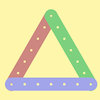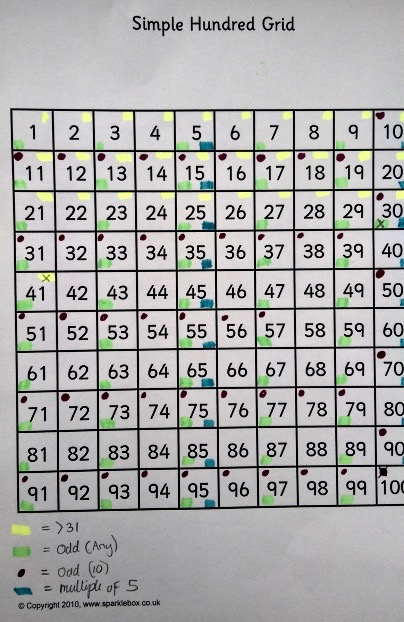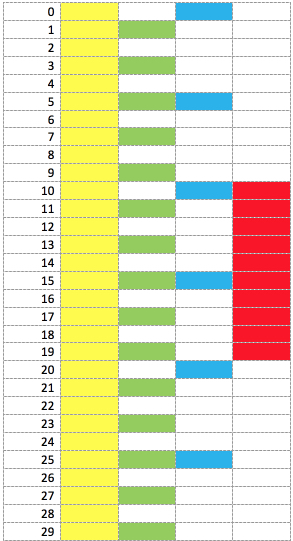#### You may also like### I'm Eight

Find a great variety of ways of asking questions which make 8.### Let's Investigate Triangles

Vincent and Tara are making triangles with the class construction set. They have a pile of strips of different lengths. How many different triangles can they make?### Noah

Noah saw 12 legs walk by into the Ark. How many creatures did he see?

# Light the Lights

##### Age 5 to 7Challenge Level
Finnan from Littletown Primary School in Devon said:Firstly, I started to write down the lights that came on for each number, starting from 1.
1 - 9 was easy to spot (Y+G or Y). After a while, though I could not see the pattern so easily.
Then I got a hundred square and used coloured pens to show when the lights came on. I put them (the lights) in the same 'order' as on the nrich page; top left = Red; top right = Yellow and so on.
Now I could spot the patterns and work out what the rules were much more quickly.
Red = any odd digit in the tens column
Yellow = any number less than 31
Green = any odd number
Blue = multiples of 5

Amy from Luckwell Primary School wrote:

Firstly, I drew a table which included all the numbers from 1-35. Then, I typed, in turn, each of the numbers in, recording which lights switched on every time. I looked for patterns and realised:
• I had recorded "blue" for each multiple of five.
• I had recorded "red" every time there was an odd number in the tens column.
• I had recorded "green" for every odd number.
• I had recorded "yellow" for every number below 30
If you typed in a negative number no lights would light up.
15 was the smallest number that would light them all up.

Ral, a teacher from Beckford Primary School in London sent us his class' thoughts:

I worked on this problem with my class. We think that the rules are:
Red: Numbers with an odd number of tens
Yellow: Numbers below 30
Green: Odd numbers
Blue: Multiples of 5 (numbers in the 5x table)

We worked it out by crossing off the numbers we used and tried to figure it out. We chose numbers we thought would match our rule. For example, blue for the x5 table and we had to choose numbers that weren't in it to see if we were correct.
Also for yellow, we had to do less than 30.
For green, we thought at first that they were odd and in the end, they were! Not forgetting red, we guessed numbers between 10 and 20 and then we found out a pattern of odd tens. We found out that yellow was 30 and we got it correct we were so overjoyed to find out we were correct.
Yellow was difficult but thanks to the wonderful class we got it right.
This was an amazing opportunity. We enjoyed it. It took half an hour!

KS1 Masters of Maths Club from the Belmont Castle Academy sent a very detailed solution:

We read the problem together and decided to try a number to see what would happen. 4 lit up only the yellow light, so we made a list of the rules it could be - even numbers, numbers in the 2x table, numbers in the 4x table or numbers less than 10.

We decided to continue finding out what rule yellow could be, so we next tried 6 as it was an even number to see if the 2x table rule could be true. The yellow light was the only one lit up again. So now we knew it couldn't be numbered in the 4x table. So then we tried 12 to check the even number rule - yellow and red lit up. So we thought yellow must be even numbers. And we made a list of rules for red as this was the first time it had come on - numbers more than 10, two-digit numbers, numbers in the 12x table.

We thought we would check to see if the rule for red was numbers 10 or more so we tried 10 - but this time yellow, red and blue lit up. So this meant that the rule couldn't be numbers 10 or more but it could be two-digit numbers. We guessed that blue could be numbers in the 10x table as this was the first time it had lit up. To test if blue came on for 10x and red was two-digit numbers we tried 100. Only blue came on. This would fit the rule, red is two-digit numbers and blue is 10x tables.

So we looked at the yellow rule again. We had said it was even numbers, so to test this we realised it should stay off for an odd number and we hadn't tried any odd numbers yet. We chose to use 9 - but yellow was still lit! And green came on too. This was the first time green had come on and the first time we had used an odd number so we thought the rule for green could be odd numbers. To try this idea, we used 15 - all the lights lit up. So now we realised blue couldn't be 10x but could be 5x.

At this point, we were losing track of which numbers met each rule and we couldn't see a pattern, so Mrs Green suggested we make a list for each light. This way we could test the rule that we had already thought of for each colour.  Under each colour we wrote the numbers we had already tested to look for a pattern - blue was the most obvious so we checked that first and it lit up for 5 and 500 so we thought that we had tried enough numbers to prove the rule 'When the number is from the 5x table the blue light will stay on'.

Next, we looked at the pattern of numbers for green - they were all odd numbers, so we tried a few random even numbers to prove the rule. The green light didn't stay on for the even numbers so now we had enough evidence to prove the rule 'When the number is odd the green light will stay on'.

Then we were left with yellow and red. We could not see an obvious pattern, so we started to write down numbers we tried that made it stay off. For yellow we noticed any large numbers we tried it hadn't come on, so thought it could be a number below 100. We tried 50, and it didn't come on. So then we thought the rule would be numbers less than 50, but when we tried 44 none of the lights came on. So we kept trying each number in order from 44 down until the yellow light came back on - it stayed on when we got to 30. We had already tried numbers less than 30 so now we had enough to prove that the rule must be 'When the number is 30 or less the yellow light stays on'.

So then we were left with the red rule. We looked at the numbers we had tried already to think of a rule. Looking at the numbers we had already tried we thought maybe it could be two-digit numbers - but then we remembered that when we tried 44 none of the lights came on. But we tried 100 to see what would happen, and the red light stayed off, but then we tried 775 and it stayed on. So again we started to write a list of numbers it stayed off for and we noticed that the tens digit was even when it turned off. We tried 98 and it stayed on. Looking at the numbers we think the final rule is 'When the number has an odd number of tens the red light stays on'.

So now we know the rules, we can work out the lowest number that will turn them all on. To turn red on, it must be an odd number of tens, which means it has to be a two-digit number. It also has to be in the 5x table to turn on blue - 10, 15, 20, 25, 30, 35... But it can't be 35 or yellow will go off. 10, 15, 30 have an odd number of tens for red, and in the 5x table for blue and are under 30 for yellow. It has to be an odd number for green so the only number it could be is 15. When we tried 15, all the lights came on.

Greg, a teacher from Australia sent us his thoughts:

I love trying to get children engaged in tackling such problems. I always try to start as if I am in kindergarten without any algebra skills so I can develop the pattern, or if I see the solution through algebra I try to decompose back to following a number pattern. Please keep up the great questions.n< 30, for n>0 then Yellow light on.
If the number is odd then the Green light turns on. 2n + 1, for n>0, then Green light on.
If the number is a multiple of 5 then the Blue light turns on. 5n, for n>0, then Blue light on.
The Red light was the most interesting, it only turns on if the number is equal to or greater than 10 and contained in a group of 10 that is odd, for example, the 10's, 30's, 50's, ”¦
10(2n + 1), for n>0, then Red Light on.
Or 20n + 10, for n >0, the Red light on.

The way the problem is presented with the red light first in our perception from left to right and working down the page made it somewhat more difficult to pick the pattern.
I saw the pattern emerge on a spreadsheet, luckily because I moved red to the end.